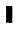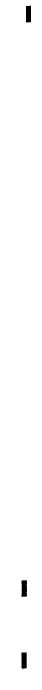Custom SearchPAR   16 DESCRIPTION  AND  OPERATION  OF CHAP  2,  SEC  II MAIN   TRANSMISSION   ASSEMBLY to   clutch   hub   assembly   35   in   such   a   way that   it   can   be   locked   to   shaft   and   carrier   2 ( f i g .    3 7 8 ,    f o l d - o u t    7 )    o r    l o c k e d    i n    s t a - tionary   position. .   O u t p u t    C l u t c h    C o m p o n e n t s    ( f i g . 377,   fold-out   6). Output   clutch   includes h u b    4 6 ,    s e v e n    i n t e r n a l - s p l i n e d    4 7 ,    a nd seven   external-splined   48   plates,   hub   as- sembly   35,   piston   assembly   40,   springs   44 and   retainer   43. H u b    4 6    i s    s p l i n e d    to s h a f t    a n d    c a r r i e r    a s s e m b l y    2    ( f i g .    3 7 8, fold-out   7).   Plates   47   (fig.   377,   fold-out 6)   have   internal   splines   which   engage   ex- ternal   splines   on   hub   46.   Plates   48   have e x t e r n a l    s p l i n e s    w h i c h    e n g a g e    i n t e r n al s p l i n e s    i n    h u b    a s s e m b l y    3 5 .    H u b    3 5    is supported   at   one   end   by   ball   bearing   95 (fig.   375,   fold-out   4)   and,   at   the   other   end by   sun   gear   assembly   52   (fig.   377,   fold-out 6),   which   rotates   on   ball   bearing   50.   Pis- ton   assembly   40   operates   in   a   bore   in   hub assembly   35.   Springs   44,   retained   by   re- t a i n e r    4 3 ,    p u s h    t h e    p i s t o n    t o    r e l e a s ed position. .   Geared   Steer   Clutch   Components ( f i g .    3 7 7 ,    f o l d - o u t    6 ) .    T h e    g e a r e d    s t e er clutch   includes   six   disks   63,   seven   plates 62,   piston   61   and   anchor   65.   The   internal splines   on   disks   63   engage   external   splines on   hub   assembly   35.   The   external   splines on   plates   62   engage   internal   splines   on anchor   65.   Piston   61   operates   in   a   bore   of t h e    t r a n s m i s s i o n    h o u s i n g    ( r i g h t    p i s t on o p e r a t e s    i n    a    b o r e    o f    t h e    e n d    c o v e r ). A n c h o r    6 5    i s    h e l d    s t a t i o n a r y    b y    b o l t s. Clutch   reaction   plate   assembly   66   serves the   steer   clutch   and   brake   jointly   and   is anchored   by   the   same   bolts   which   retain anchor d. 65.   - Operation   of   Geared   Steer Planetary,   Output   Clutch   and Geared   Steer   Clutch   (figs.   374. 3 7 7 ,    3 7 8 ,    f o l d - o u t s    3 ,    6 ,    7 ) ( 1 )    P l a n e t a r y    g e a r i n g    a n d    t w o clutches   operate   in   two   ways   to   transmit rotation   to   output   shaft   18   (fig.   374,   fold- o u t    3 ) .    U n d e r    o n e    c o n d i t i o n ,    t o r q u e    is transmitted   at   a   1   to   1   ratio.   Under   the other   condition,   there   is   a   speed   reduction [from   transfer   driven   gear   shaft   4   (fig. 380,   fold-out   9)   to   output   shaft   18   (fig. 374,   fold-out   3)]   of   1.477   to   1.   The   right and   left   systems   have   identical   and   simul- taneous   functions   during   straight   travel   of t h e     v e h i c l e . T h e y     o p e r a t e     s e p a r a t e ly during   steer. (2)   During   straight   travel   in   all gears,   except   first   and   reverse   1,   drive   is direct.   In   first   gear   forward   and   reverse 1,   there   is   a   speed   reduction   through   the system.   This   applies   to   the   right   and   left out   puts   simultaneously   during   straight travel.   During   steer,   drive   is   transmitted to   one   output   while   the   other   output   is e i t h e r    s t o p p e d    o r    s l o w e d. T h e    v e h i c le steers   toward   the   stopped   or   slowed   side. (3)   Two   methods   of   steering   are employed   —  clutch-brake   steer   (first,   sec- ond   and   reverse   1   gears)   and   geared   steer ( t h i r d ,    f o u r t h    a n d    r e v e r s e    2    g e a r s ) .    In clutch   brake   steer,   one   output   drives   while the   other   is   disengaged   and   braked.   In g e a r e d    s t e e r ,    o n e    o u t p u t    i s    s l o w e d    b y s p e e d    r e d u c t i o n    i n    t h e    s t e e r    p l a n e t a ry while   the   other   output   continues   to   drive at   1   to   1   ratio.   Thus,   during   geared   steer, t h e    r i g h t-    a n d    l e f t - o u t p u t    c l u t c h e s    a nd geared   steer   clutches   operate   singly.   A geared   steer   clutch   is   applied   at   one   side of   the   transmission   while   an   output   clutch is   applied   at   the   opposite   side. ( 4 )    W h e n    h y d r a u l i c    p r e s s u r e    is applied   to   the   output   clutch,   the   piston a s s e m b l y    4 0    ( f i g .    3 7 7 ,    f o l d - o u t    6 )    c o m- p r e s s e s    p l a t e s    4 7    a n d    4 8    a g a i n s t    t h e  reaction   plate   surface   of   sun   gear   assem- bly   52.   This   locks   sun   gear   52   and   shaft and   carrier   assembly   2   (fig.   378,   fold-out 7)   together.   Ring   gear   14   then   drives   the carrier   at   1   to   1   ratio.   When   hydraulic pressure   is   applied   to   the   geared   steer c l u t c h ,    p i s t o n    6 1    ( f i g .    3 7 7 ,    f o l d - o u t    6) compresses   plates   62   and   disks   63   against reaction   plate   assembly   66.   This   locks   sun gear   assembly   52   stationary.   Ring   gear   14 (fig.   378,   fold-out   7)   then   drives   shaft   and c a r r i e r    a s s e m b l y    2 ,    a t    r e d u c e d    s p e e d, around   the   stationary   sun   gear. Speed r e d u c t i o n    ( f r o m    o u t p u t    t r a n s f e r    d r i v en gear   to   transmission   output   coupling)   is 1.477   to   1.   When   either   clutch   is   released, springs   push   its   piston   to   released   position. Change  2  17